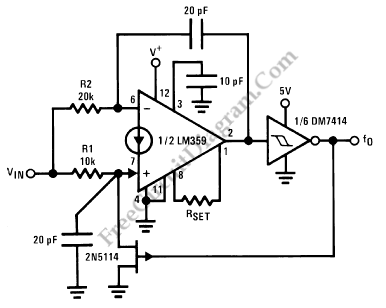## Opto-Isolated-Input 4-Digit Voltmeter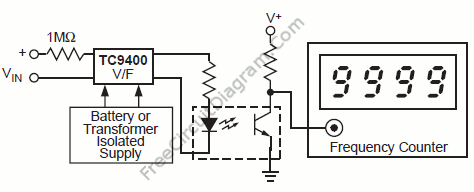The voltage that control a V/F converters will be displayed if we use a frequency counter, which is directly proportional to the input voltage. A 1 second time base will give 4 digit resolution with 1 reading per second when the V/F converters is running at 10KHz full scale. To transmit the frequency, we can use the optoisolator, so there […]

## 13-Bit A/D Converter with TC9400 VFC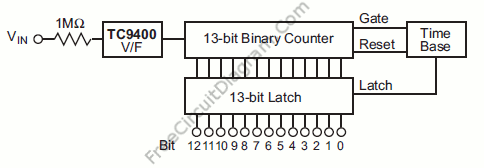If we don’t need a very fast analog-to-digital conversion (ADC), by combining TC9400 V/F converter with a counter, latch and time base, we can build a13 bit binary A/D converter. A 1 second time base will provide one conversion per second when the V/F converter is set up for 10KHz full scale. The high input impedance of  TC9400 make it […]

## A/D Conversion with Voltage-to-Frequency Converter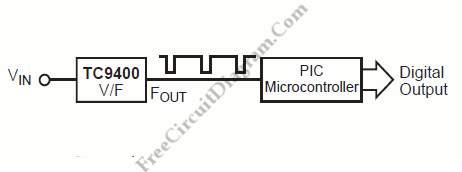To accomplish A/D conversion with V/F converter in a microcontroller system, there are two schemes that can be utilized. First, VIN is measured by counting the FOUT frequency for 1ms, 10ms, 100ms, or 1 second depending on the number of digits of resolution required. The final count is then directly proportional to VIN. (The microcontroller provides the time base) .Second, […]

## Temperature Measurement (Sensing) and Monitoring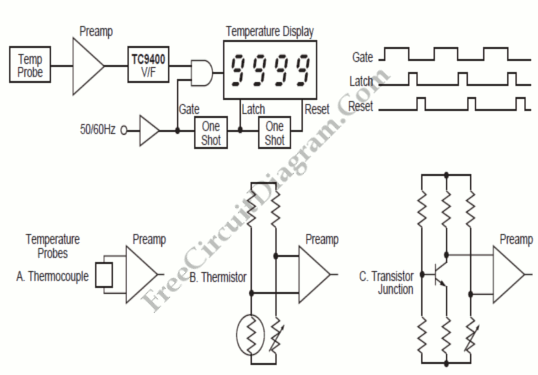Temperature  measurement (sensing) and monitoring will be economical and straightforward if we apply the input of  TC9400 V/F converter with a voltage from output of a probe, such as shown in diagram below. The V/F output is simply counted to display the temperature. The TC9400 can be used to modulate an RF transmitter for a long distance data transmission. The […]

## TC9400 10Hz to 10kHz Voltage-to-Frequency Converter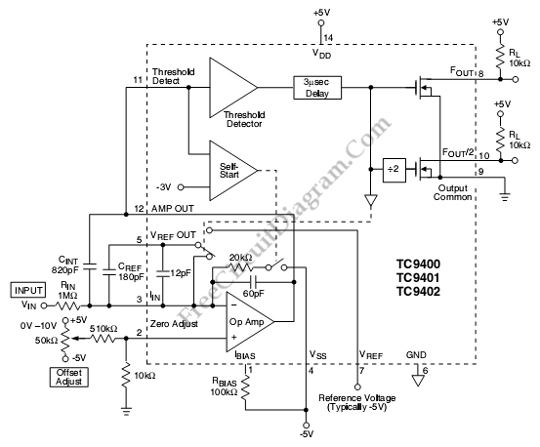This is a TC9400 10Hz to 10kHz Voltage-to-Frequency Converter circuit. This ciruit uses TC9400 to convert voltage to frequency. The TC9400 uses charge balancing principal. This circuit uses input resistor to convert the input voltage to input current. Then the capacitor will change the current into charge which will decrease the Op amp output voltage linearly. This circuit also featured […]

## 555 – 741 IC Voltage To Frequency Converter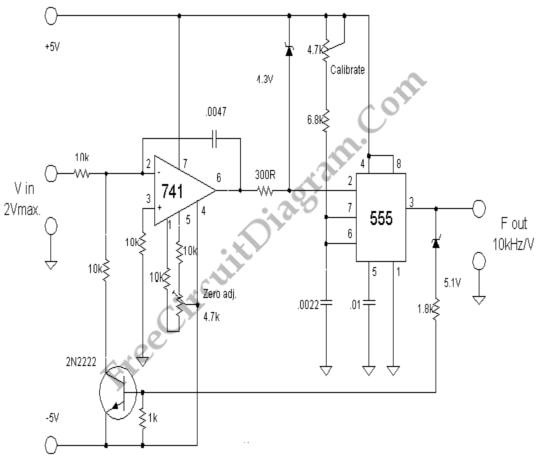This voltage to frequency converter (VFC) circuit uses 555 IC and 741 op-amp as the main components. Up to 20kHz oscillation can be produced by this circuit. Here is the schematic diagram: The zero adjustment is used to adjust the lowest frequency, short the input to ground and adjust this pot to obtain the lowest frequency limit. This circuit uses […]